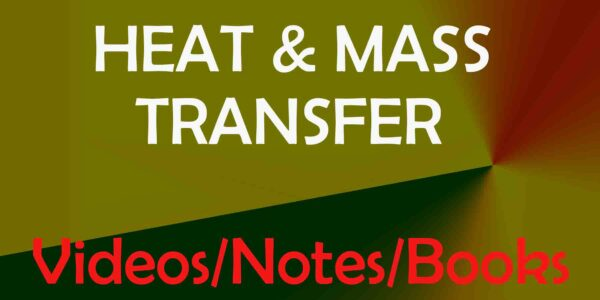heat and mass transfer pdf | HMT notes pdf |Handwritten notes hmt | Heat & mass transfer Notes| Mechanical Engineering Notes | Written by Ashish SirHeat and Mass Transfer is a peer-reviewed scientific journal published by Springer. It serves the circulation of new developments in the field of basic research of heat and mass transfer phenomena, as well as related material properties and their measurements. Thereby applications to engineering problems are promoted.

Heat and Mass Transfer notes Syllabus

Unit-1 Basic Concepts: Modes of heat transfer, Fourier’s law, Newton’s law, Stefan Boltzman law; thermal resistance and conductance, analogy between flow of heat and electricity, combined heat transfer process; Conduction: Fourier heat conduction equation, its form in rectangular, cylindrical and spherical coordinates, thermal diffusivity, linear one dimensional steady state conduction through a slab, tubes, spherical shells and composite structures, electrical analogies, critical-insulation-thickness for pipes, effect of variable thermal conductivity.

Unit 2 Extended surfaces (fins): Heat transfer from a straight and annular fin (plate) for a uniform cross section; error in measurement of temperature in a thermometer well, fin efficiency, fin effectiveness, applications; Unsteady heat conduction: Transient and periodic conduction, heating and cooling of bodies with known temperatures distribution, systems with infinite thermal conductivity, response of thermocouples.

Unit 3 Convection: Introduction, free and forced convection; principle of dimensional analysis, Buckingham ‘pie’ theorem, application of dimensional analysis of free and forced convection, empirical correlations for laminar and turbulent flow over flat plate and tubular geometry; calculation of convective heat transfer coefficient using data book.

Unit 4 Heat exchangers: Types- parallel flow, counter flow; evaporator and condensers, overall heat transfers coefficient, fouling factors, log-mean temperature difference (LMTD), method of heat exchanger analysis, effectiveness of heat exchanger, NTU method; Mass transfer: Fick’s law, equi-molar diffusion, diffusion coefficient, analogy with heat transfer, diffusion of vapour in a stationary medium.

Unit 5 Thermal radiation: Nature of radiation, emissive power, absorption, transmission, reflection and emission of radiation, Planck’s distribution law, radiation from real surfaces; radiation heat exchange between black and gray surfaces, shape factor, analogical electrical network, radiation shields.

Boiling and condensation: Film wise and drop wise condensation; Nusselt theory for film wise condensation on a vertical plate and its modification for horizontal tubes; boiling heat transfer phenomenon, regimes of boiling, boiling correlations.

ChAPTER-1

Unit-1 Basic Concepts: Modes of heat transfer, Fourier’s law, Newton’s law, Stefan Boltzman law; thermal resistance and conductance, analogy between flow of heat and electricity, combined heat transfer process; Conduction: Fourier heat conduction equation, its form in rectangular, cylindrical and spherical coordinates, thermal diffusivity, linear one dimensional steady state conduction through a slab, tubes, spherical shells and composite structures, electrical analogies, critical-insulation-thickness for pipes, effect of variable thermal conductivity.